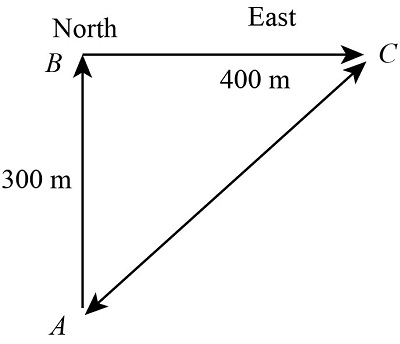# A car travels 300 m [N] along a road, then turns a corner and drives an additional 400 m [E]....

## Question:

A car travels 300 m N along a road, then turns a corner and drives an additional 400 m E. Draw a vector diagram of the path traveled by the car, and then calculate the car's distance traveled and its displacement.

## Distance and displacement:

The total value of the distance traveled between the initial point and the final point is known as the distance traveled by the object. The displacement is the shortest distance traveled by the object between the initial point and the final point in the coordinate axis is known as the displacement of the body.

Given data

• The value of the distance {eq}AB = 300\;{\rm{m}} {/eq}
• The value of the length {eq}BC = 400\;{\rm{m}} {/eq}

The vector diagram of the problem is shown below.The value of the distance travelled is

{eq}d = AB + BC {/eq}

Substitute the value in the above equation

{eq}\begin{align*} d &= 300 + 400\\ &= 700\;{\rm{m}} \end{align*} {/eq}

The value of the displacement travelled is

{eq}d = \left( {\sqrt {A{B^2} + B{C^2}} } \right) {/eq}

Substitute the value in the above equation

{eq}\begin{align*} d &= \left( {\sqrt {{{300}^2} + {{400}^2}} } \right)\\ &= 500\;{\rm{m}} \end{align*} {/eq}

Thus the value of the distance is {eq}700\;{\rm{m}} {/eq} and the value of the displacement is {eq}500\;{\rm{m}} {/eq}